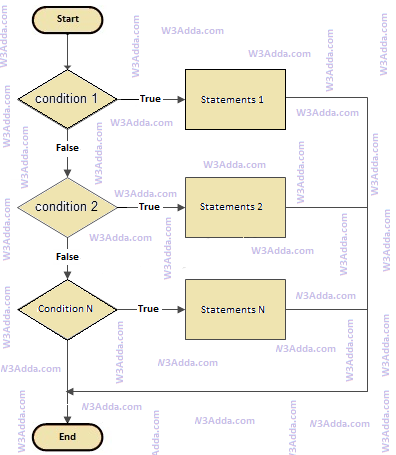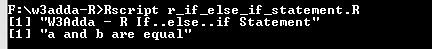# R if else if Statement

In this tutorial you will learn about the R if else if Statement and its application with practical example.

## R if else if Statement

In R, if..else..if statement allows us add alternative set of test conditions in if..else statement using else if and single else statements for if condition. In such way if..else..if statement is used to select one among several blocks of code to be executed.

## R if..else..if Statement Flow Diagramr-if-else-if

Syntax:-

Example:-

Output:-In this tutorial we have learn about the R if else if Statement and its application with practical example. I hope you will like this tutorial.